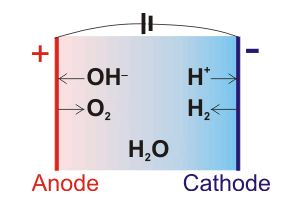# What Is Electrolysis Of Water? - Chemistry Question

The electrolysis is defined as the process by which ionic substances are decomposed into simpler substances when an electric current is passed through them.

### Uses of Electrolysis

• Electrolysis is done for coating one metal on another.
• The industrial use includes various metals such as aluminium, magnesium, chlorine, and fluorine etc.

## Water electrolysis

Electrolysis of water is the chemical reaction in which the decomposition of hydrogen and oxygen gases is carried by passing an electric current. The electrolysis of water is mainly carried out to yield pure hydrogen and oxygen gases. It involves passing an electric current through the water which results in the decomposition of water into hydrogen and oxygen.

Following is the equation of electrolysis of water:

Half reactions in the electrolysis of pure water at pH=7, and at 25°Care

At cathode: 2H2O(l) + 2e → H2(g) + 2OH   E° = -0.42 V

At anode: 2H2O → O2(g) + 4H+ + 4e    E° = +0.82 V

The net reaction of electrolysis of water is given as;

2H2O + Electrical energy → O2 + 2H2      E° = -1.24 V

The number of hydrogen molecules produced is thus twice the number of oxygen molecules. After electrolysis of water, hydrogen is collected at negative chose and oxygen is collected at the positive anode. Hydrogen is double in volume than oxygen.(152)(38)